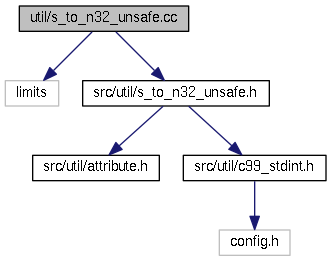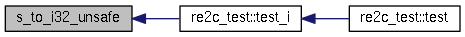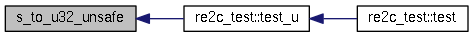src
s_to_n32_unsafe.cc File Reference
`#include <limits>`
`#include "src/util/s_to_n32_unsafe.h"`
Include dependency graph for s_to_n32_unsafe.cc:Go to the source code of this file.

## Functions

bool s_to_u32_unsafe (const char *s, const char *s_end, uint32_t &number)

bool s_to_i32_unsafe (const char *s, const char *s_end, int32_t &number)

## Function Documentation

 bool s_to_i32_unsafe ( const char * s, const char * s_end, int32_t & number )

Definition at line 25 of file s_to_n32_unsafe.cc.

26 {
27  int64_t i = 0;
28  if (*s == '-')
29  {
30  ++s;
31  for (; s != s_end; ++s)
32  {
33  i *= 10;
34  i -= *s - 0x30;
35  if (i < std::numeric_limits<int32_t>::min())
36  {
37  return false;
38  }
39  }
40  }
41  else
42  {
43  for (; s != s_end; ++s)
44  {
45  i *= 10;
46  i += *s - 0x30;
47  if (i > std::numeric_limits<int32_t>::max())
48  {
49  return false;
50  }
51  }
52  }
53  number = static_cast<int32_t> (i);
54  return true;
55 }

Here is the caller graph for this function:bool s_to_u32_unsafe ( const char * s, const char * s_end, uint32_t & number )

Definition at line 7 of file s_to_n32_unsafe.cc.

8 {
9  uint64_t u = 0;
10  for (; s != s_end; ++s)
11  {
12  u *= 10;
13  u += static_cast<uint32_t> (*s) - 0x30;
14  if (u >= std::numeric_limits<uint32_t>::max())
15  {
16  return false;
17  }
18  }
19  number = static_cast<uint32_t> (u);
20  return true;
21 }

Here is the caller graph for this function: